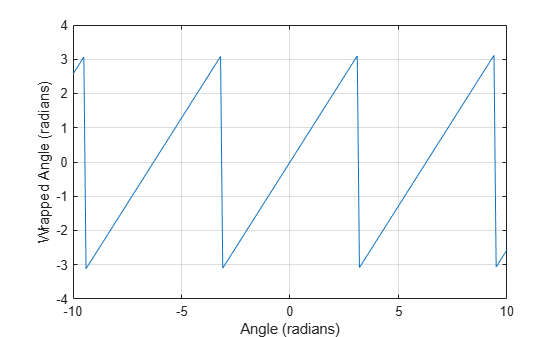# wrapToPi

Wrap angle in radians to [−pi pi]

## Syntax

``lambdaWrapped = wrapToPi(lambda)``

## Description

example

````lambdaWrapped = wrapToPi(lambda)` wraps angles in `lambda`, in radians, to the interval `[−pi, pi]` such that `pi` maps to `pi` and `−pi` maps to `−pi`. In general, odd, positive multiples of `pi` map to `pi` and odd, negative multiples of `pi` map to `−pi`.```

## Examples

collapse all

Specify a short list of angles to wrap.

`lambda = [-2*pi -pi-0.1 -pi -2.8 3.1 pi pi+1 2*pi];`

Wrap the angles to the range [-$\pi$, $\pi$] radians.

`lambdaWrapped = wrapToPi(lambda)`
```lambdaWrapped = 1×8 0 3.0416 -3.1416 -2.8000 3.1000 3.1416 -2.1416 0 ```

Specify a second list of angles, and wrap them.

```lambda2 = -10:0.1:10; lambda2Wrapped = wrapToPi(lambda2);```

Plot the wrapped angles. The wrapped angles stay in the range [-$\pi$, $\pi$] radians.

```plot(lambda2,lambda2Wrapped) xlabel("Angle (radians)") ylabel("Wrapped Angle (radians)") grid on```## Input Arguments

collapse all

Angles, specified as a numeric vector.

Data Types: `single` | `double` | `int8` | `int16` | `int32` | `int64` | `uint8` | `uint16` | `uint32` | `uint64` | `logical`

## Output Arguments

collapse all

Wrapped angles, specified as a numeric vector with values in the range `[−pi, pi]`.

## Extended Capabilities

### C/C++ Code GenerationGenerate C and C++ code using MATLAB® Coder™.